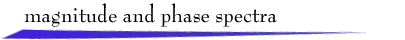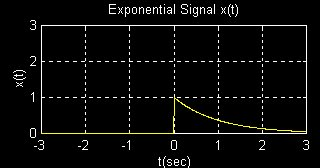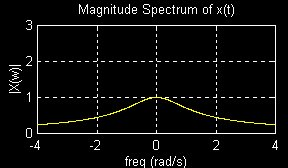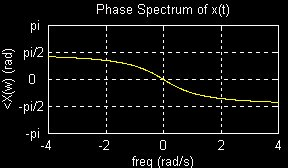## Introduction:

Important frequency characteristics of a signal x(t) with Fourier transform X(w) are displayed by plots of the magnitude spectrum, |X(w)| versus w, and phase spectrum, <X(w) versus w.

This demonstration uses the one-sided, real, decaying (b > 0) exponential signal

x(t) = ae-btu(t)
which has Fourier transform
X(w) = a/(b + jw)
The magnitude spectrum of the signal is
|X(w)| = |a|/(b2 + w2) 1/2
The phase spectrum is (in radians)
<X(w) = tan-1(w/b), if a > 0
<X(w) = p + tan-1(w/b), if a < 0

 A downloadable zip-file for execution on Matlab is available here. This permits interactive exploration of properties of spectra using various example signals. If you are familiar with the transformations below, go directly to the zip-file.

Plots of the exponential signal x(t) = e-tu(t) (a=1, b=1) and the corresponding spectra are shown below:Amplitude Scale y(t) = ax(t) = ae-tu(t)

Time Shift y(t) = x(t - to) = e-(t - to) u(t - to)

Time Scale y(t) = x(bt) = e-btu(bt) = e-btu(t)

Time Truncation y(t) = x(t) = e-tu(t) for |t| < T, and y(t) = 0 for |t| > T

Also, you can select transformations on the spectra, i.e. on the Fourier transform, and view the effects on the signal:

Frequency Scale Y(w) = X(cw)

Ideal Filtering Y(w) = X(w) for |w| < W, and Y(w) = 0 for |w| > Wreturn to demonstrations page